# 1. In the following graph, a firm's short run total cost curve is given as ABCD...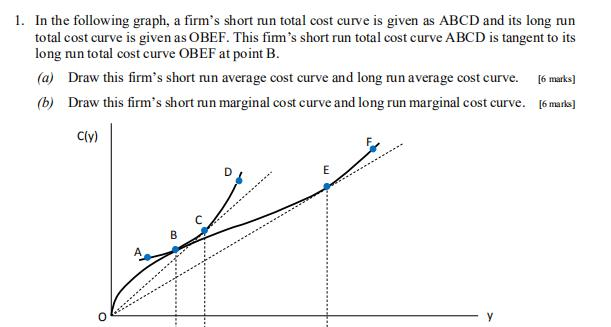1. In the following graph, a firm's short run total cost curve is given as ABCD and its long run total cost curve is given as OBEF. This fimm's short run total cost curve ABCD is tangent to its long run total cost curve OBEF at point B. (a) Draw this firm's short run average cost curve and long run average cost curve. [6 marks] (b) Draw this firm's short run marginal cost curve and long run marginal cost curve. [6 marks]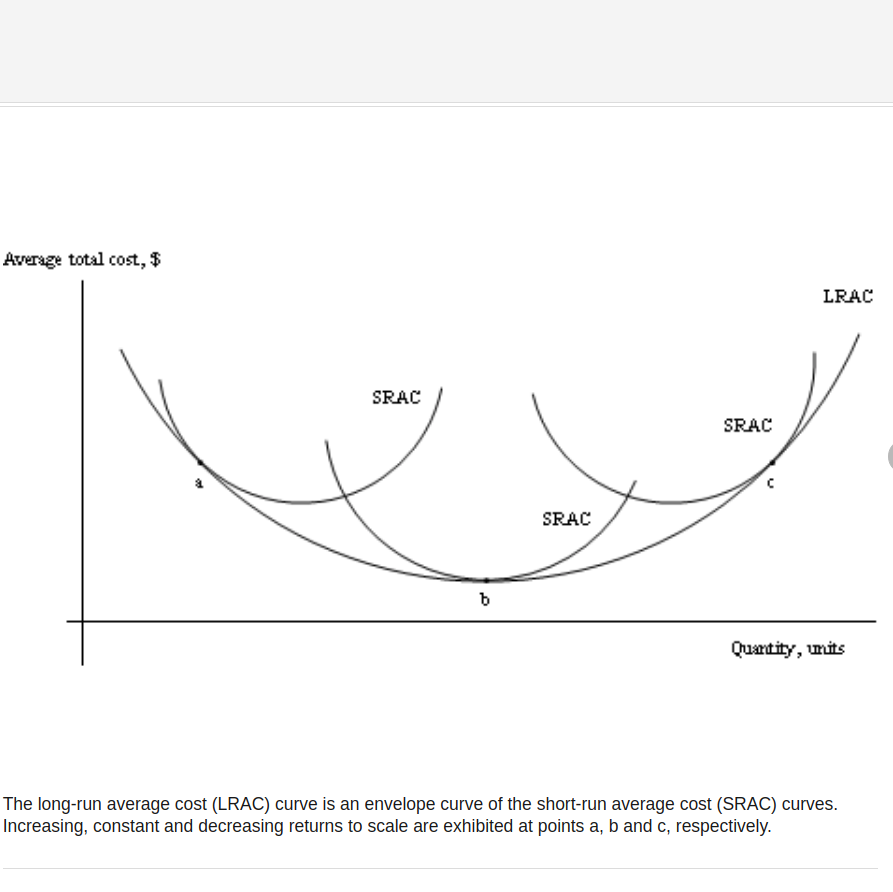We know that as per the Cost Analysis Theory, the long run average cost curve is derived mostly from the minimum points of the short run average cost curves, because of which the long run average cost curve is also called as Envelop Curve that is tangent to the each and every short run average curve at some point. The points a, b and c represents th stages of increasing, constant and decreasing return to scale respectively.

If the given Short run Tatal Cost Curve is derived at the time period of Increasing return to scale, then the Short run average cost which is tangent to Long run average cost curve at point "a", then SRAC1 will be the required short run average cost curve along with the obtained LRAC for the given details.

If the given Short run Tatal Cost Curve is derived at the time period of Constant return to scale, then the Short run average cost which is tangent to Long run average cost curve at point "b", then SRAC2 will be the required short run average cost curve along with the obtained LRAC for the given details.

If the given short run Tatal Cost Curve is derived at the time period of Decreasing return to scale, then the Short run average cost which is tangent to Long run average cost curve at point "c", then SRAC3 will be the required short run average cost curve along with the obtained LRAC for the given details.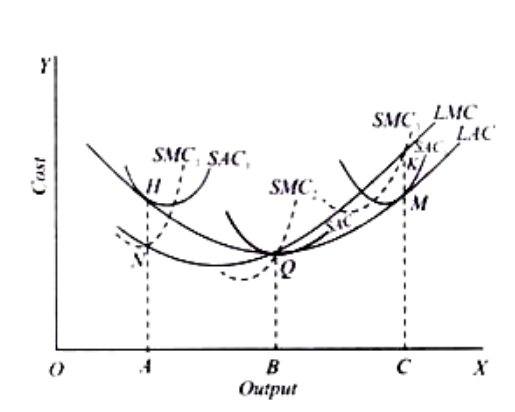According to Cost Analysis Theory, Shrort run Marginal Cost curve (SRMC curve) passes through the minimum point of the Short run average cost curve (SRAC curve). and also that the Long run Marginal Cost curve (LRMC curve) passes through the minimum point of th of the Long run Average Cost curve (LRAC curve) where the LRAC curve is tangent to the SRAC curve that too only when there is constant return to scale is the operating phenomina.

If the given Short run Tatal Cost Curve is derived at the time period of Increasing return to scale, then the Short run Marginal cost curve (SRMC1) which is passing through the minimum point of SRAC1 will be the required short run marginal cost curve along with the obtained LRMC curve for the given details.

If the given Short run Tatal Cost Curve is derived at the time period of constant return to scale, then the Short run Marginal cost curve (SRMC2) which is passing through the minimum point of SRAC2 will be the required short run marginal cost curve along with the obtained LRMC curve for the given details.

If the given Short run Tatal Cost Curve is derived at the time period of decreasing return to scale, then the Short run Marginal cost curve (SRMC3) which is passing through the minimum point of SRAC3 will be the required short run marginal cost curve along with the obtained LRMC curve for the given details.

#### Earn Coin

Coins can be redeemed for fabulous gifts.

Similar Homework Help Questions
• ### The following graph shows the short-run average total cost curves and the long-run average total cost...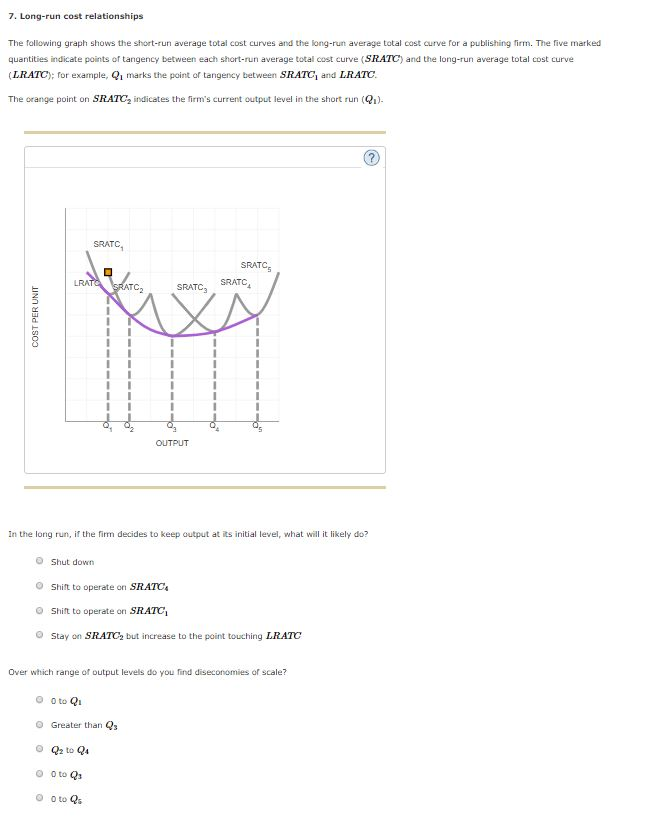The following graph shows the short-run average total cost curves and the long-run average total cost curve for a publishing firm. The five marked quantities indicate points of tangency between each short-run average total cost curve ( SRATC ) and the long-run average total cost curve ( LRATC ); for example, Q1 marks the point of tangency between SRATC1 and LRATC . 7. Long-run cost relationships The following graph shows the short-run average total cost curves and the long-run average...

• ### The following graph shows the short-run average total cost curves and the long-run average total cost...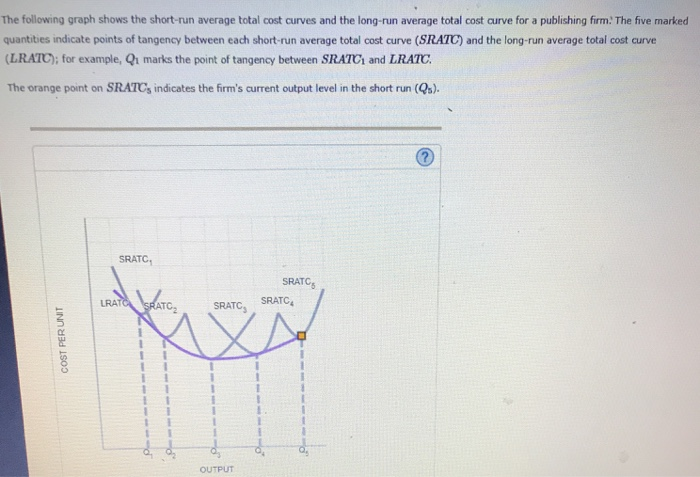The following graph shows the short-run average total cost curves and the long-run average total cost curve for a publishing firm. The five marked quantities indicate points of tangency between each short-run average total cost curve (SRATC) and the long-run average total cost curve (LRATC); for example, Qı marks the point of tangency between SRATC1 and LRATC The orange point on SRATC, indicates the firm's current output level in the short run(Q). SRATC, SRATCE SRATC SRATC, SRATC COST PERUNT OUTPUT...

• ### Long-run cost relationships The following graph shows the short-run average total cost curves and the long-run average total cost curve for a publishing firm.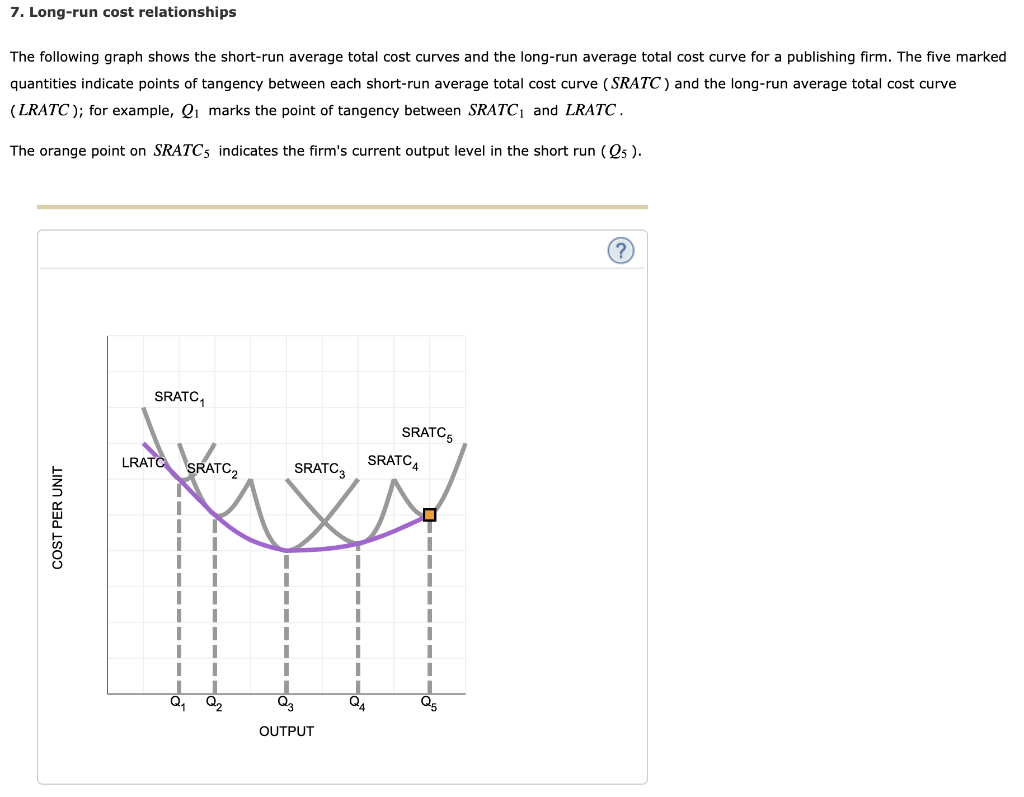7. Long-run cost relationships The following graph shows the short-run average total cost curves and the long-run average total cost curve for a publishing firm. The five marked quantities indicate points of tangency between each short-run average total cost curve (SRATC) and the long-run average total cost curve (LRATC); for example, Q1 marks the point of tangency between SRATC1 and LRATC The orange point on SRATCs indicates the firm's current output level in the short run (Q5). SRATC SRATC SRATC4...

• ### The following graph shows the short-run average total cost curves and the long-run average total cost...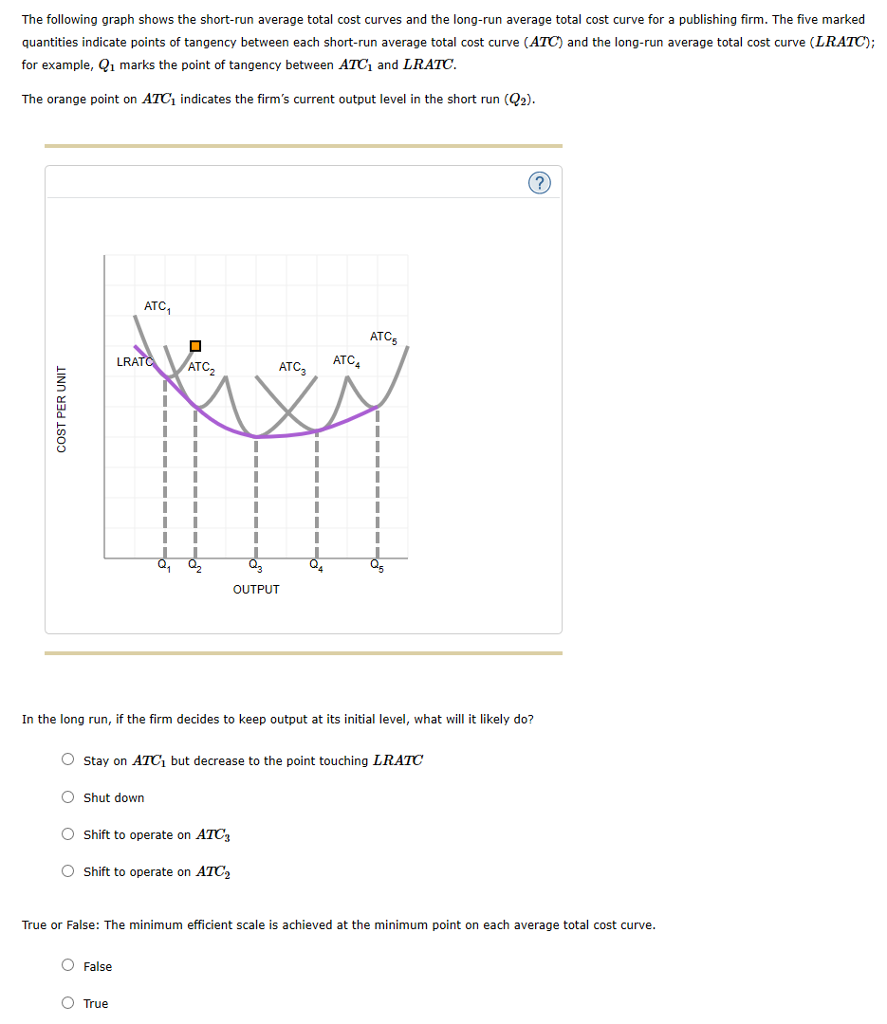The following graph shows the short-run average total cost curves and the long-run average total cost curve for a publishing firm. The five marked quantities indicate points of tangency between each short-run average total cost curve (ATC) and the long-run average total cost curve (LRATC); for example, Qı marks the point of tangency between ATCi and LRATC The orange point on ATC1 indicates the firm's current output level in the short run (2) ATC, ATCs ATC ATC OUTPUT In the...

• ### The following graph shows the short run total cost curves and the long tun total cost...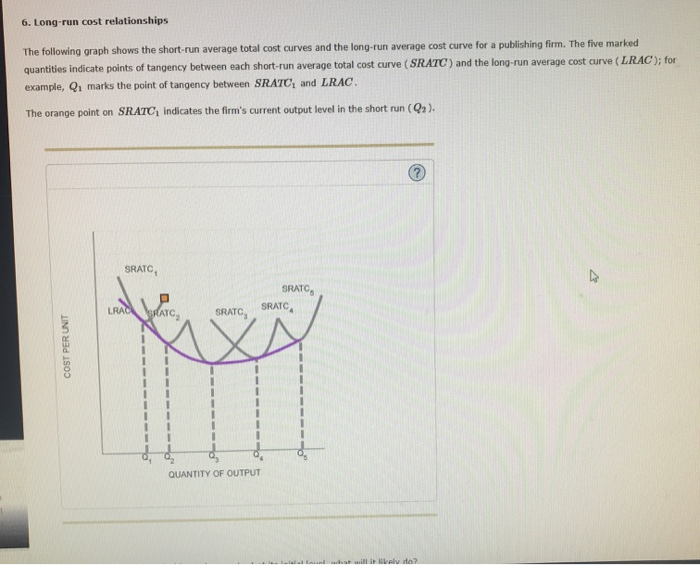The following graph shows the short run total cost curves and the long tun total cost curves for a publishing firm. the five marked quantities indicate points of tangency between each short run average total cost curve and the long run average cost curve. could someone please help me to answer this and give a little explenation for my similar problems? 6. Long-run cost relationships The following graph shows the short-run average total cost curves and the long-run average cost...

• ### 10. Long-run cost relationships The following graph shows the short-run average total cost curves and the...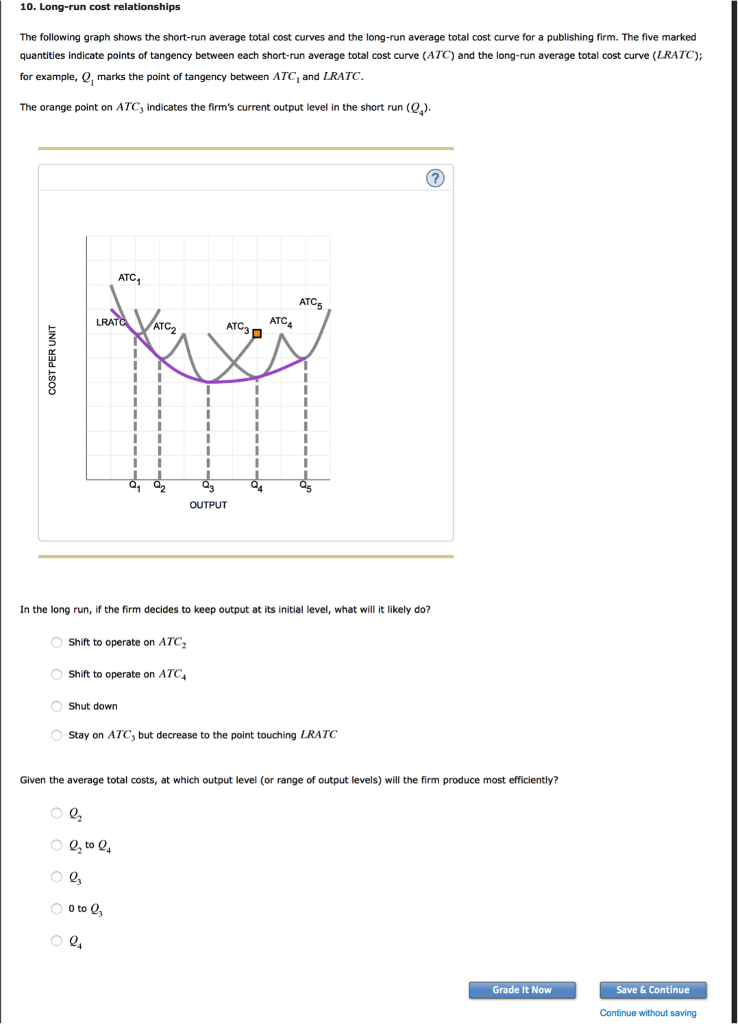10. Long-run cost relationships The following graph shows the short-run average total cost curves and the long-run average total cost curve for a publishing firm. The five marked quantities indicate points of tangency between each short-run average total cost curve (ATC) and the long-run average total cost curve (LRATC); for example, Q, marks the point of tangency between ATC, and LRATC. The orange point on ATC3 indicates the firm's current output level in the short run (0). ATC AT LRA...

• ### 6. Long-run cost relationships The following graph shows the short-run average total cost curves and the...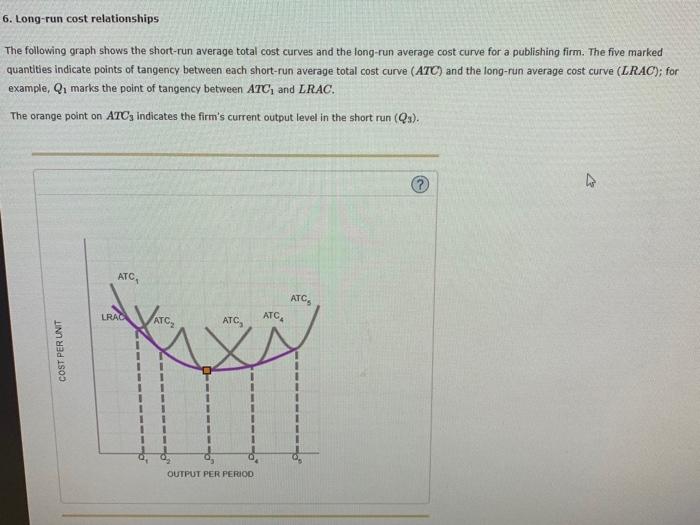6. Long-run cost relationships The following graph shows the short-run average total cost curves and the long-run average cost curve for a publishing firm. The five marked quantities indicate points of tangency between each short-run average total cost curve (ATC) and the long-run average cost curve (LRAC); for example, Q1 marks the point of tangency between ATC, and LRAC. The orange point on ATC indicates the firm's current output level in the short run (Qs). ATC LRAC ATC ATC, COST...

• ### P M C ATC Av In the graph above, MC is the firm's marginal cost curve, ATC is the firm's average total cost curve,...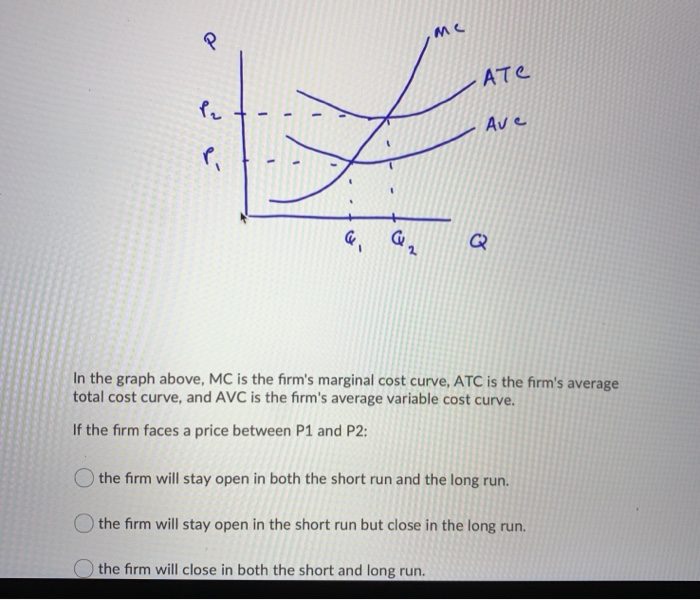P M C ATC Av In the graph above, MC is the firm's marginal cost curve, ATC is the firm's average total cost curve, and AVC is the firm's average variable cost curve. If the firm faces a price between P1 and P2: the firm will stay open in both the short run and the long run. the firm will stay open in the short run but close in the long run. the firm will close in both the short...

• ### In the graph above, MC is the firm's marginal cost curve, ATC is the firm's average...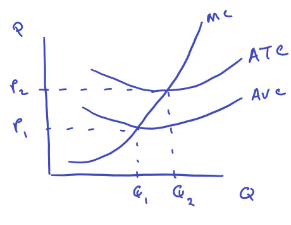In the graph above, MC is the firm's marginal cost curve, ATC is the firm's average total cost curve, and AVC is the firm's average variable cost curve. If the firm faces a price between P1 and P2: the firm will stay open in both the short run and the long run. the firm will stay open in the short run but close in the long run. the firm will close in both the short and long run. - -...

• ### In the graph above, MC is the firm's marginal cost curve, ATC is the firm's average...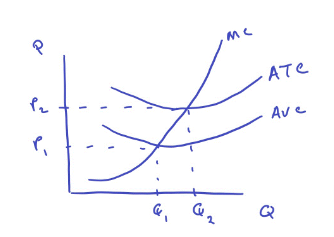In the graph above, MC is the firm's marginal cost curve, ATC is the firm's average total cost curve, and AVC is the firm's average variable cost curve. If the firm faces a price greater than P2, then the firm will stay open in both the short and the long run. the firm will stay open in the short run, but close in the long run. the firm will close in both the short and the long run. Ате 12...

Free Homework App